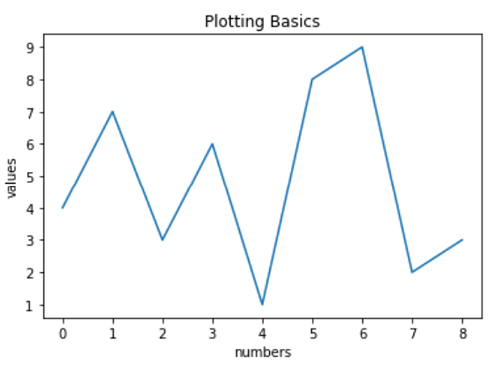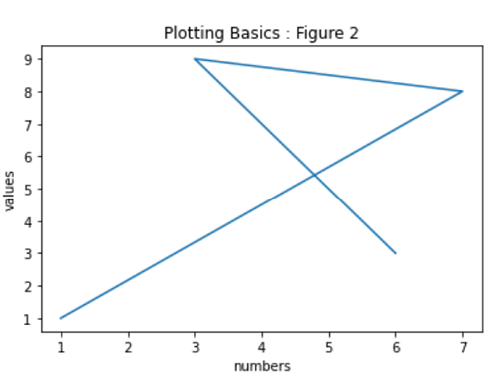# Python | Pyplot in Matplotlib

Python | Pyplot in Matplotlib: In this tutorial, we are going to learn about the pyplot which is a matplotlib module with the example.
Submitted by Anuj Singh, on July 04, 2020

matplotlib.pyplot provides a number of different functions that can be used to plot and visualize our data. It also gives a free hand to do different operations with a plot such as annotations, marking, etc. In this article, we are going to learn a very basic plot type and how to label its axis and furthermore will add the Figure title.Plotting using a single array automatically puts it on the y-axis. So adding two arrays in order (x-axis, y-axis) can produce our desired plot. One example is shown in figure 2.## Python code to demonstrate example of Pyplot in Matplotlib

```# Data Visualization using Python
# Basics of Plotting

import matplotlib.pyplot as plt

# plotting using plt.pyplot()
plt.plot([4,7,3,6,1,8,9,2,3])

# axis labeling
plt.xlabel('numbers')
plt.ylabel('values')

# figure name
plt.title('Plotting Basics')

##########################################

# plotting using plt.pyplot() Figure 2
plt.plot([1,7,3,6],[1,8,9,3])

# axis labeling
plt.xlabel('numbers')
plt.ylabel('values')

# figure name
plt.title('Plotting Basics : Figure 2')
```